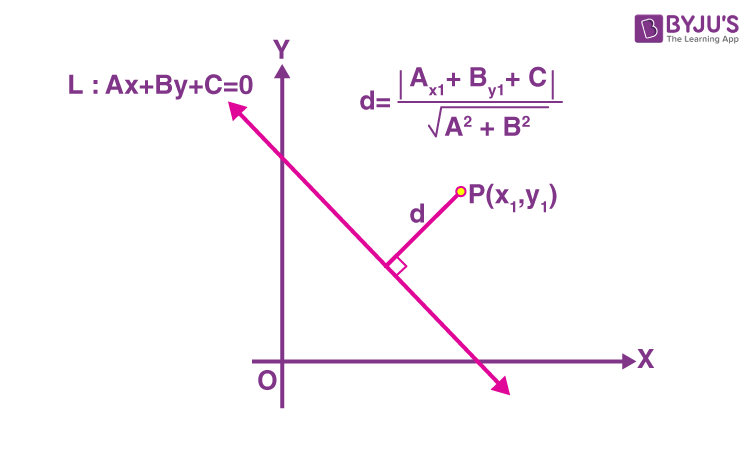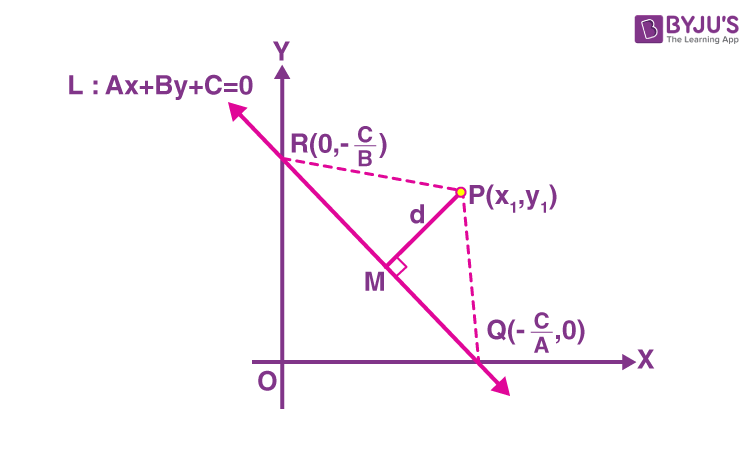Checkout JEE MAINS 2022 Question Paper Analysis : Checkout JEE MAINS 2022 Question Paper Analysis :

# Distance of a Point From a Line

In geometry, the distance of a point from a line is the shortest distance between the point and the line. In general, we can draw an infinite number of lines from a point to a line in a plane. Now the question is how to measure the distance between this point and line, which is the shortest distance from a point to a line. The only possibility to get the shortest distance is to draw a perpendicular line segment on the line through the given point. This is because joining the point and line with more than one line provides us with a triangle.

## What is Distance of a Point From a Line?

The shortest between a point and a line is the distance between them. It measures the minimum distance or length required to move a point on the line.

As we know, in a triangle, we can find the height by constructing an altitude. Hence, we have to draw a perpendicular so that we can form a right triangle here. Also, the longest side in a right triangle is the hypotenuse. Therefore, if we draw the foot of the perpendicular from the point to the line and any other segment joining the point to the line, we get a right triangle. However, the second line segment drawn here will always be the hypotenuse of the right triangle formed. Now, we can find the distance between the given point and line using different formulas, which are explained in the next section.## Perpendicular Distance of a Point From a Line

In this section, you will learn how to derive the formula for the distance of a point from a line mathematically.

As defined above, the distance more specifically, the shortest distance of a point from a line is the length of the perpendicular drawn from the point to the line. Below are the steps to derive the formula for finding the shortest distance between a point and line.

Step 1: Consider a line L : Ax + By + C = 0 whose distance from the point P (x1, y1) is d.

Step 2: Draw a perpendicular PM from the point P to the line L as shown in the figure below.

Step 3: Let Q and R be the points where the line meets the x-and y-axes, respectively.

Step 4: Coordinates of the points can be written as Q(-C/A, 0) and R(0, -C/B).## Derivation of Distance of a Point From a Line

Let’s derive the formula using the formulas of the area of the triangle in different aspects.

In triangle PQR,

ar(ΔPQR) = (1/2) × Base × Height = (1/2) × PM × QR

⇒ PM = [2 ar(ΔPQR)]/2….(i)

In coordinate geometry, the area of triangle with vertices (x1, y1), (x2, y2) and (x3, y3) is

Δ = (1/2) |x1(y2 – y3) + x2(y3 – y1) + x3(y1 – y2)|

Here,

(x1, y1) = P(x1, y1)

(x2, y2) = Q(-C/A, 0)

(x3, y3) = R(0, -C/B)

Now,

ar(ΔPQR) = (1/2) |x1(0 + C/B) + (-C/A)(-C/B – y1) + 0(y1 – 0)|

ar(ΔPQR) = (1/2) |x1(C/B) + (C2/AB) + y1(C/A)|

⇒ 2 ar(ΔPQR) = |C/AB| . |Ax1 + By1 + C|….(ii)

Distance QR = √[(0 + C/A)2 + (C/B – 0)2]

= √[(C2/A2) + (C2/B2)]

Let’s find QR using the distance formula.

QR = |C/AB| √(A2 + B2)….(iii)

Substituting (ii) and (iii) in (i),

PM = [|C/AB| . |Ax1 + By1 + C|] / [|C/AB| √(A2 + B2)]

d = |Ax1 + By1 + C|]/ √(A2 + B2)

Therefore, the perpendicular distance (d) of a line Ax + By+ C = 0 from a point (x1, y1) is given by

d = |Ax1 + By1 + C|]/ √(A2 + B2)Go through the example given below to understand the above process and how to apply the formula to solve mathematical problems based on the distance between point and line.

## Solved Examples

Question 1:

Find the distance of the point (-3, 5) from the line 4x – 3y – 26 = 0.

Solution:

Given equation of a line is: 4x – 3y – 26 = 0

Point: (-3, 5)

Comparing these with the standard forms,

A = 4, B = -3, C = -26

x1 = -3, y1 = 5

We know that the perpendicular distance (d) of a line Ax + By+ C = 0 from a point (x1, y1) is given by

d = |Ax1 + By1 + C|]/ √(A2 + B2)

Substituting the values,

d = |4(-3) + (-3)(5) + (-26)|/√[(4)2 + (-3)2]

= |-12 – 15 – 26|/√(16 + 9)

= |-53|/√25

= 53/5

Hence, the required distance is 53/5 units.

Question 2:

Find the distance between the point (5,1) and the line y = 3x + 1.

Solution: Given point (5,1) and line y = 3x + 1.

We need to find the distance between them.

y = 3x + 1

3x + 1 – y = 0

Comparing these with the standard forms,

A = 3, B = -1, C = 1

x1 = 5, y1 = 1

We know that the perpendicular distance (d) of a line Ax + By+ C = 0 from a point (x1, y1) is given by

d = |Ax1 + By1 + C|]/ √(A2 + B2)

Substituting the values,

d = [|3.5 + (-1)(1) + 1|]/√(32 + (-1)2)

d = 15/√10